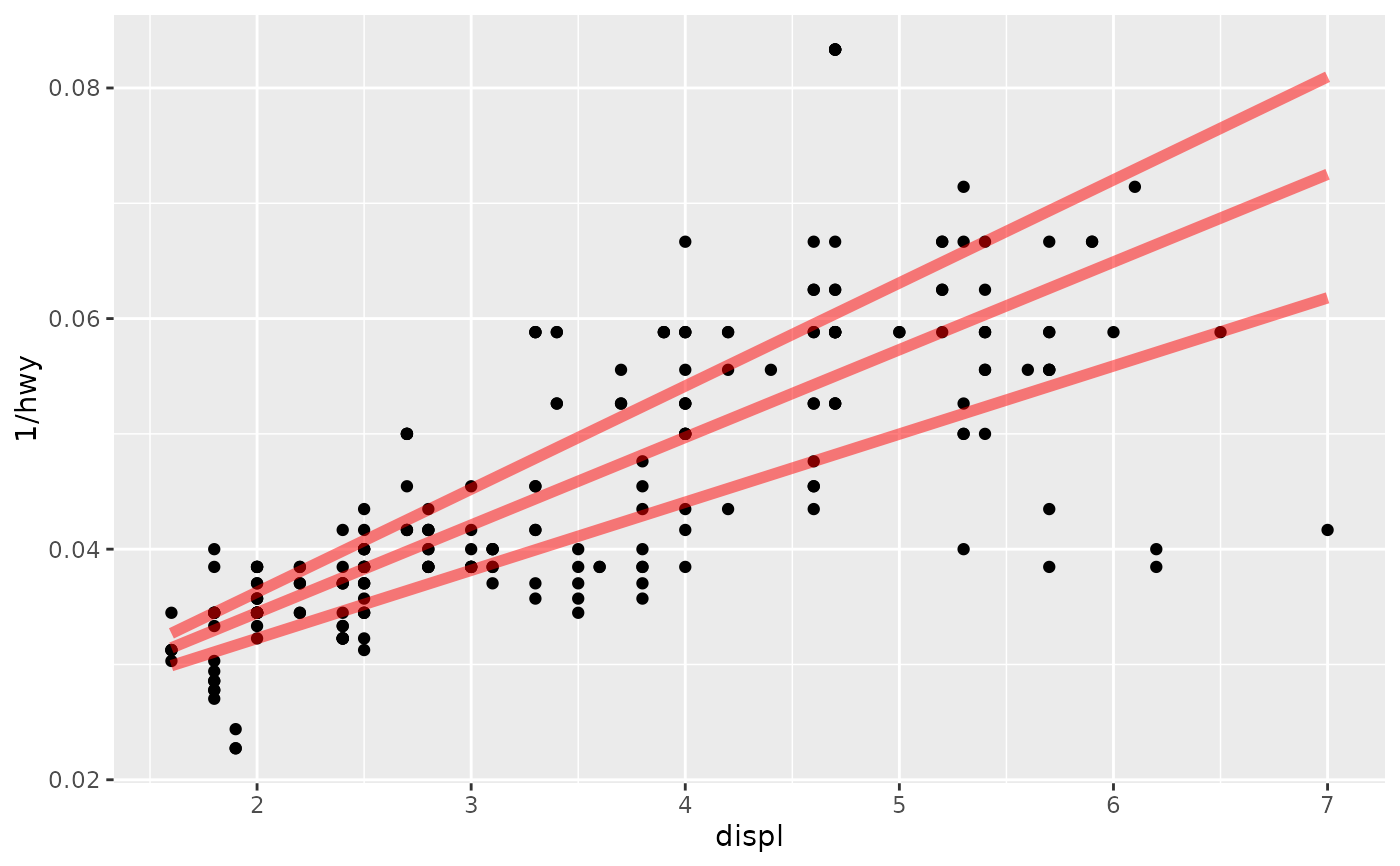This fits a quantile regression to the data and draws the fitted quantiles with lines. This is as a continuous analogue to geom_boxplot().

## Usage

geom_quantile(
mapping = NULL,
data = NULL,
stat = "quantile",
position = "identity",
...,
lineend = "butt",
linejoin = "round",
linemitre = 10,
na.rm = FALSE,
show.legend = NA,
inherit.aes = TRUE
)

stat_quantile(
mapping = NULL,
data = NULL,
geom = "quantile",
position = "identity",
...,
quantiles = c(0.25, 0.5, 0.75),
formula = NULL,
method = "rq",
method.args = list(),
na.rm = FALSE,
show.legend = NA,
inherit.aes = TRUE
)

## Arguments

mapping

Set of aesthetic mappings created by aes(). If specified and inherit.aes = TRUE (the default), it is combined with the default mapping at the top level of the plot. You must supply mapping if there is no plot mapping.

data

The data to be displayed in this layer. There are three options:

If NULL, the default, the data is inherited from the plot data as specified in the call to ggplot().

A data.frame, or other object, will override the plot data. All objects will be fortified to produce a data frame. See fortify() for which variables will be created.

A function will be called with a single argument, the plot data. The return value must be a data.frame, and will be used as the layer data. A function can be created from a formula (e.g. ~ head(.x, 10)).

position

Position adjustment, either as a string naming the adjustment (e.g. "jitter" to use position_jitter), or the result of a call to a position adjustment function. Use the latter if you need to change the settings of the adjustment.

...

Other arguments passed on to layer(). These are often aesthetics, used to set an aesthetic to a fixed value, like colour = "red" or size = 3. They may also be parameters to the paired geom/stat.

lineend

Line end style (round, butt, square).

linejoin

Line join style (round, mitre, bevel).

linemitre

Line mitre limit (number greater than 1).

na.rm

If FALSE, the default, missing values are removed with a warning. If TRUE, missing values are silently removed.

show.legend

logical. Should this layer be included in the legends? NA, the default, includes if any aesthetics are mapped. FALSE never includes, and TRUE always includes. It can also be a named logical vector to finely select the aesthetics to display.

inherit.aes

If FALSE, overrides the default aesthetics, rather than combining with them. This is most useful for helper functions that define both data and aesthetics and shouldn't inherit behaviour from the default plot specification, e.g. borders().

geom, stat

Use to override the default connection between geom_quantile() and stat_quantile().

quantiles

conditional quantiles of y to calculate and display

formula

formula relating y variables to x variables

method

Quantile regression method to use. Available options are "rq" (for quantreg::rq()) and "rqss" (for quantreg::rqss()).

method.args

List of additional arguments passed on to the modelling function defined by method.

## Aesthetics

geom_quantile() understands the following aesthetics (required aesthetics are in bold):

• x

• y

• alpha

• colour

• group

• linetype

• linewidth

• weight

Learn more about setting these aesthetics in vignette("ggplot2-specs").

## Computed variables

These are calculated by the 'stat' part of layers and can be accessed with delayed evaluation.

• after_stat(quantile)
Quantile of distribution.

## Examples

m <-
ggplot(mpg, aes(displ, 1 / hwy)) +
geom_point()
m + geom_quantile()
#> Smoothing formula not specified. Using: y ~ x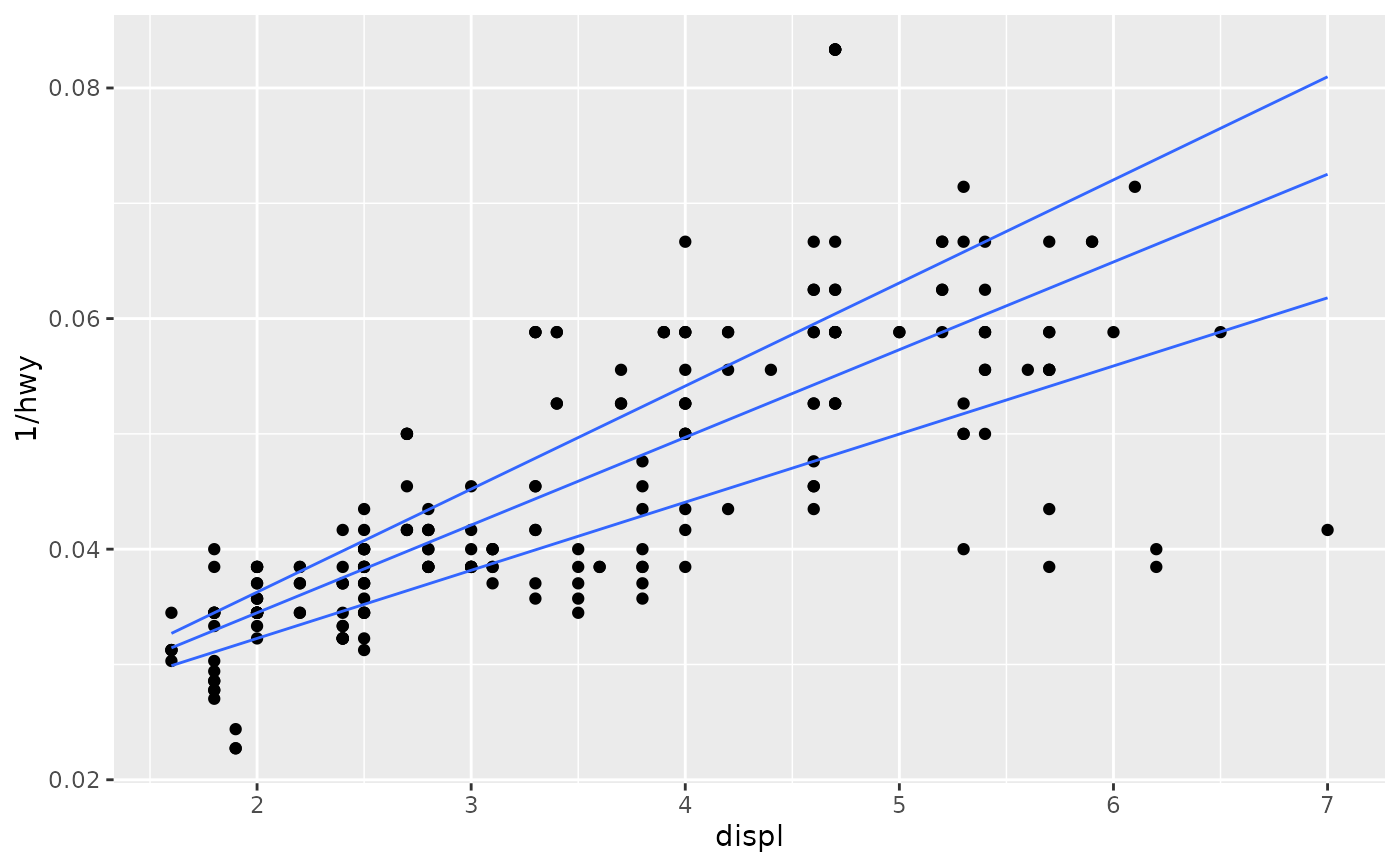m + geom_quantile(quantiles = 0.5)
#> Smoothing formula not specified. Using: y ~ x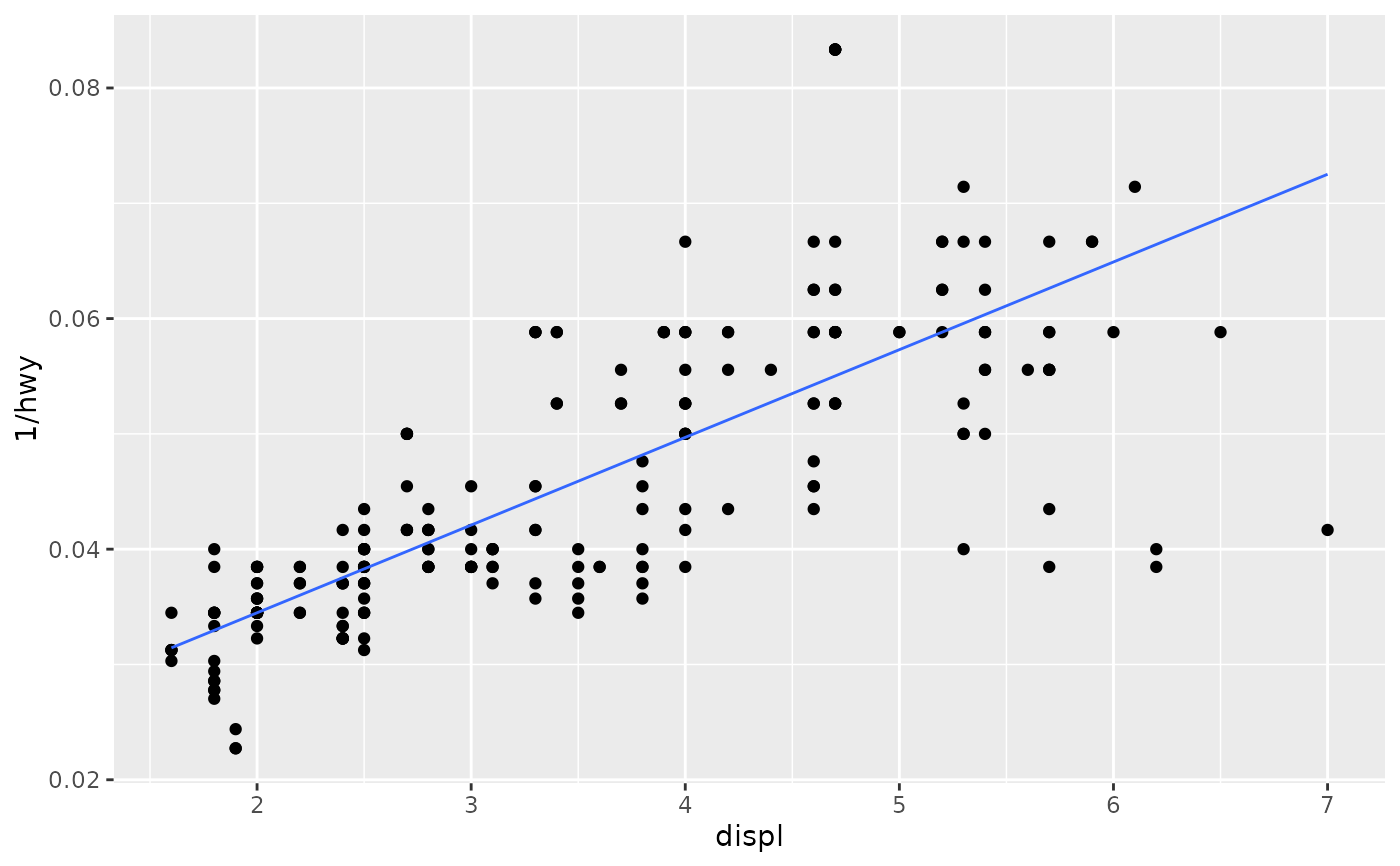q10 <- seq(0.05, 0.95, by = 0.05)
m + geom_quantile(quantiles = q10)
#> Smoothing formula not specified. Using: y ~ x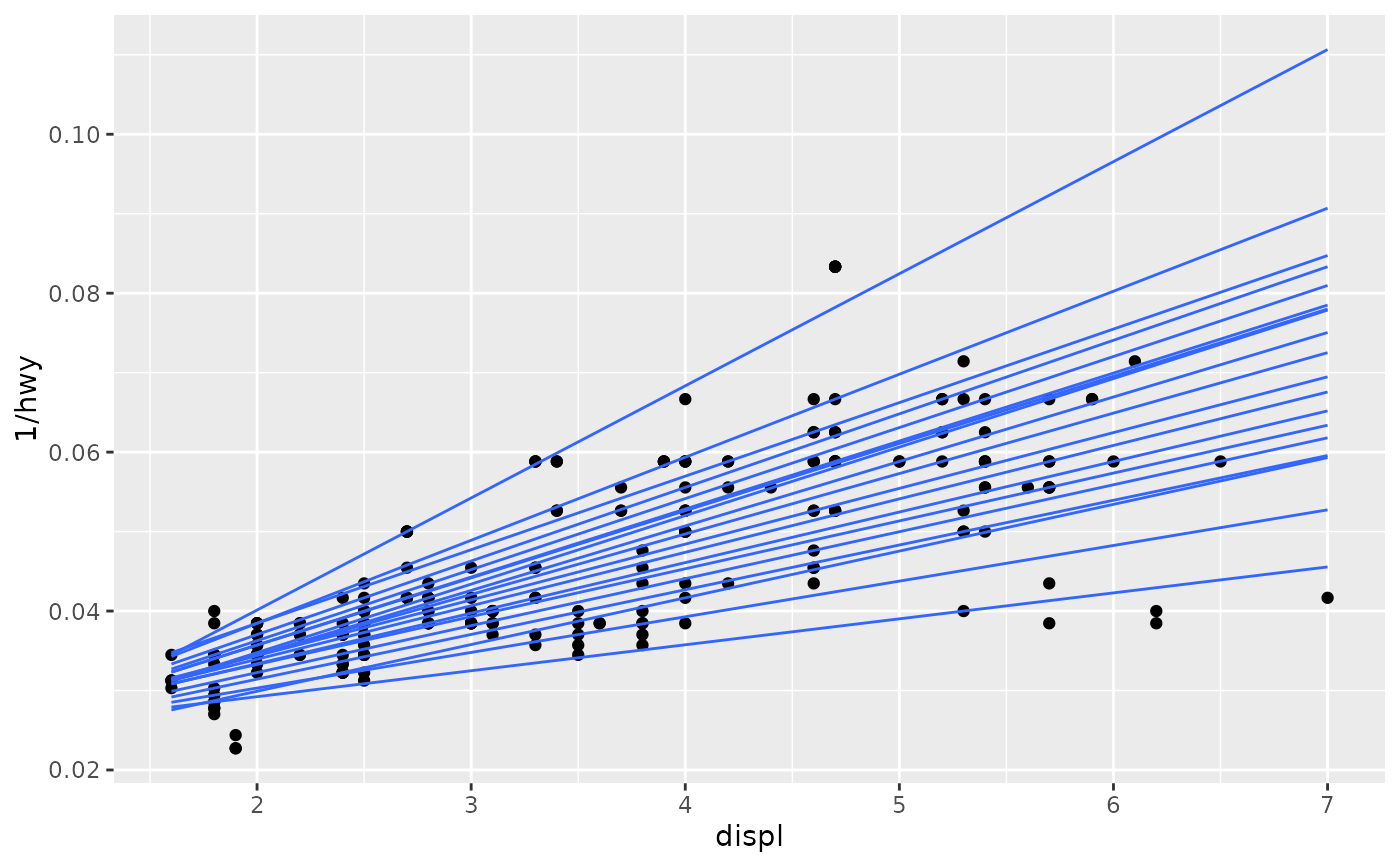# You can also use rqss to fit smooth quantiles
m + geom_quantile(method = "rqss")
#> Smoothing formula not specified. Using: y ~ qss(x, lambda = 1)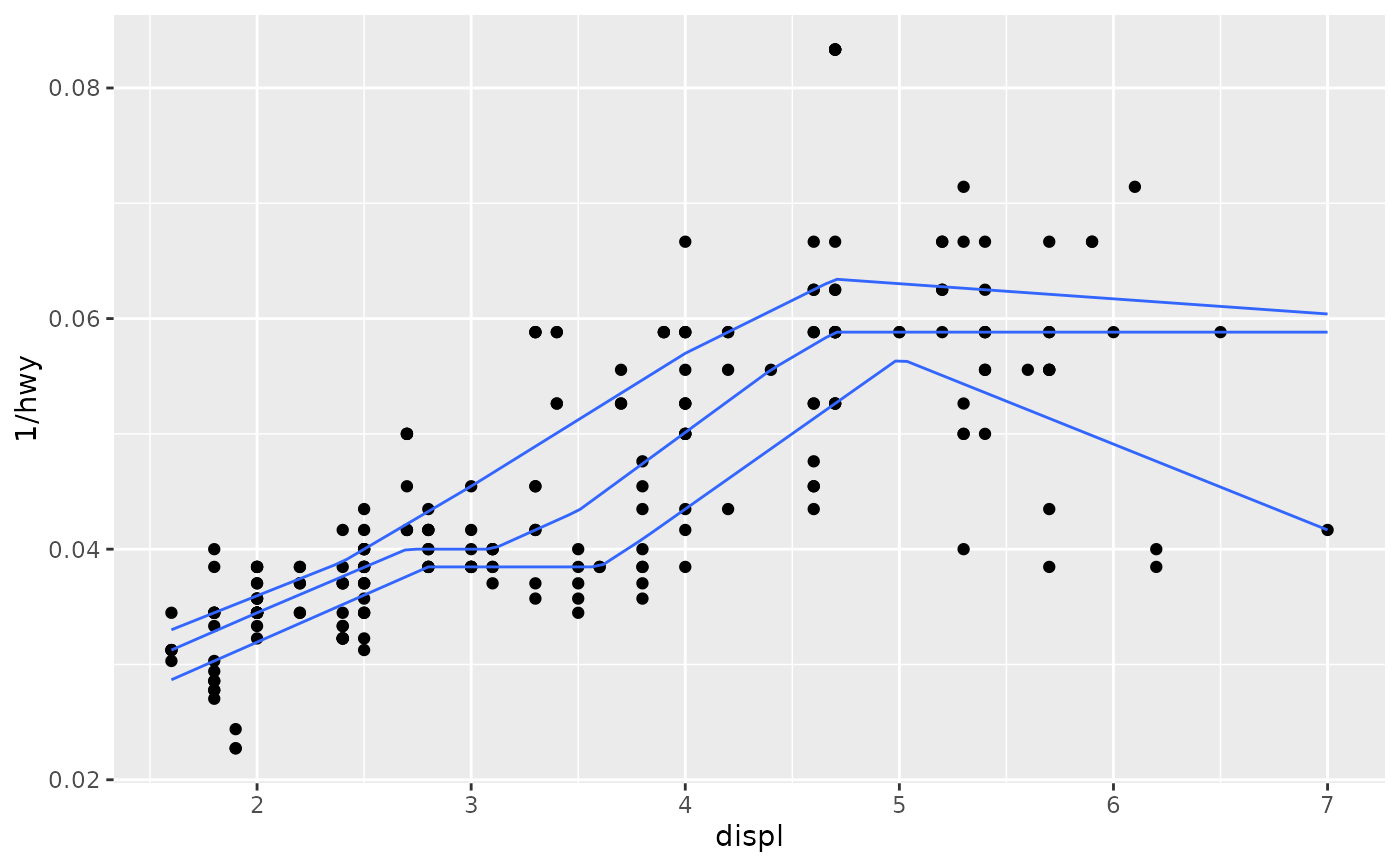# Note that rqss doesn't pick a smoothing constant automatically, so
# you'll need to tweak lambda yourself
m + geom_quantile(method = "rqss", lambda = 0.1)
#> Smoothing formula not specified. Using: y ~ qss(x, lambda = 0.1)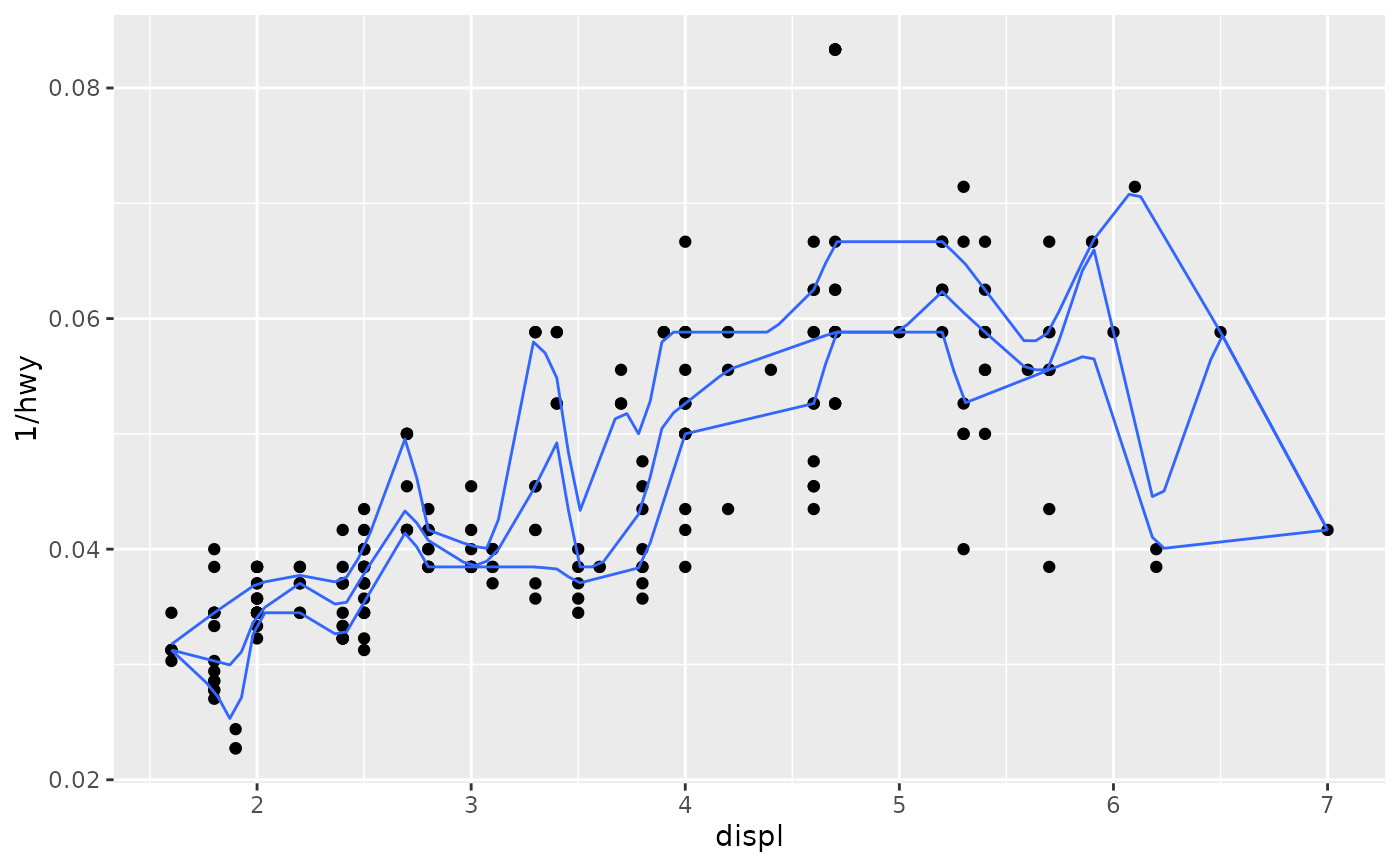# Set aesthetics to fixed value
m + geom_quantile(colour = "red", linewidth = 2, alpha = 0.5)
#> Smoothing formula not specified. Using: y ~ x